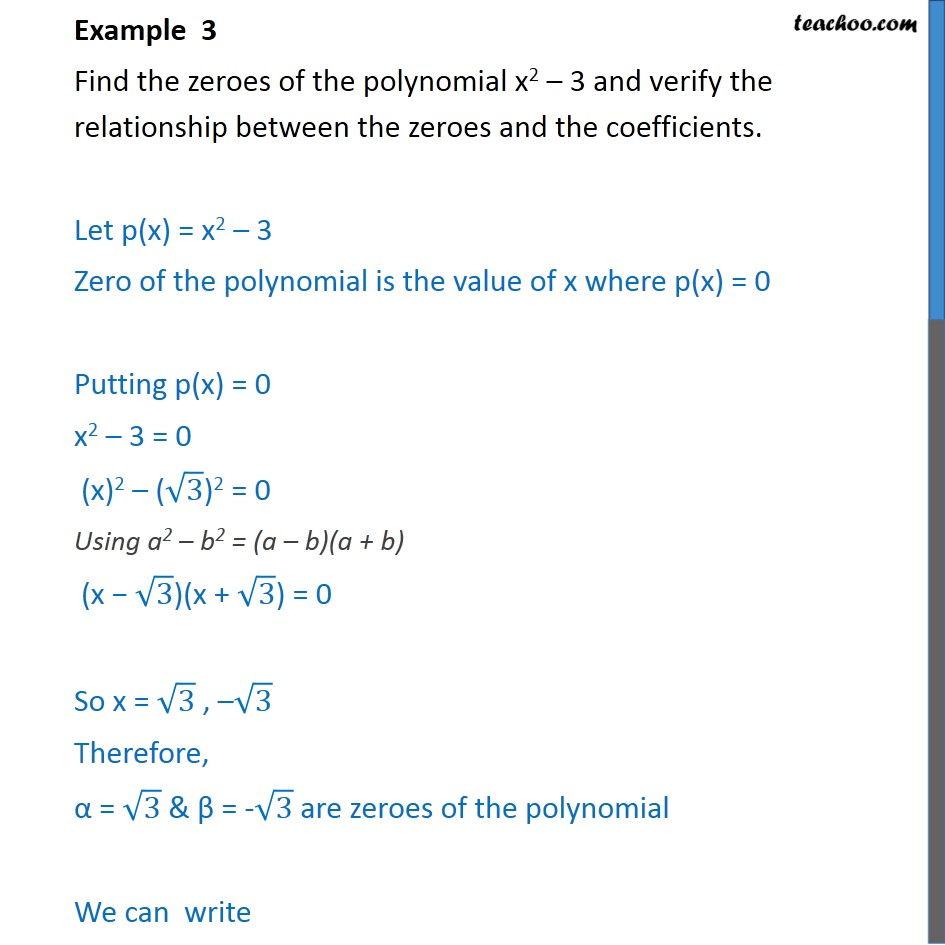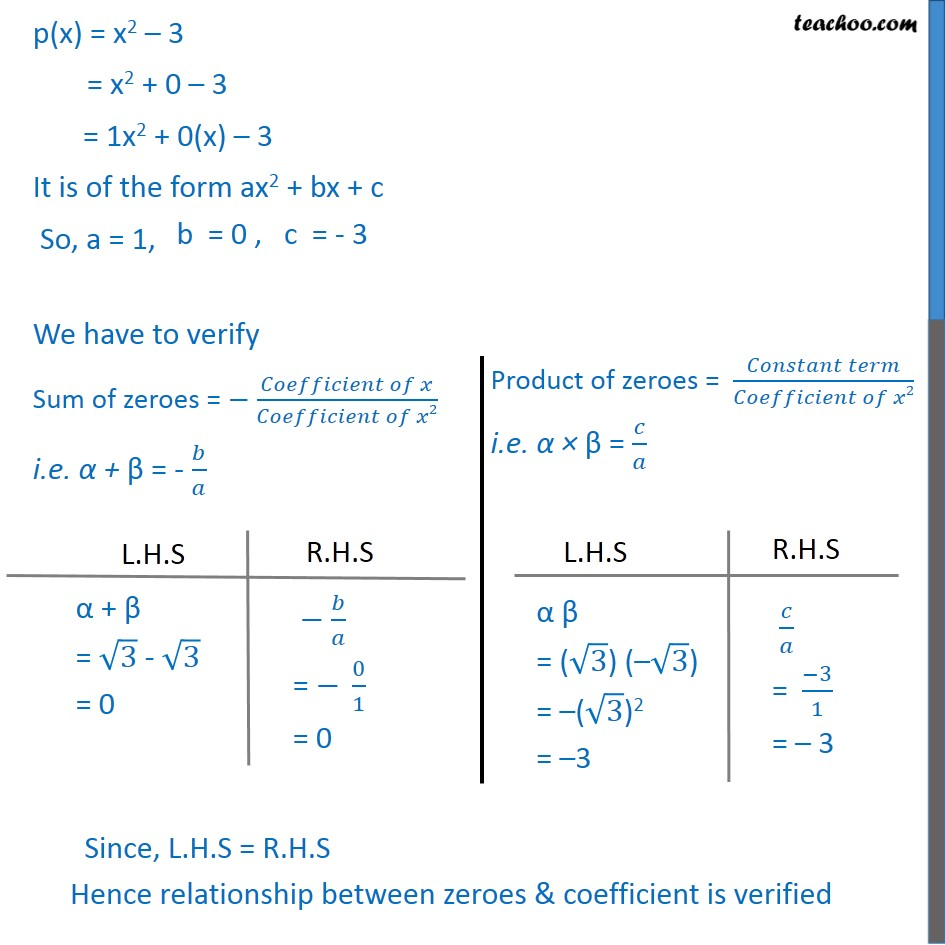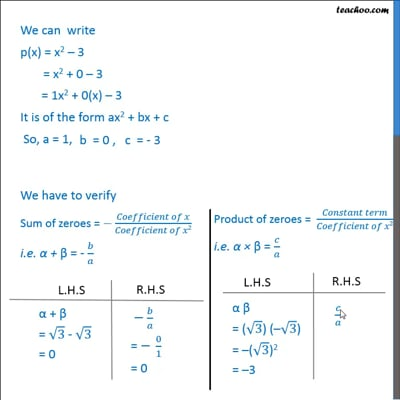Examples

Chapter 2 Class 10 Polynomials (Term 1)
Serial order wiseThis video is only available for Teachoo black users

### Transcript

Example 3 Find the zeroes of the polynomial x2 3 and verify the relationship between the zeroes and the coefficients. Let p(x) = x2 3 Zero of the polynomial is the value of x where p(x) = 0 Putting p(x) = 0 x2 3 = 0 (x)2 ( 3)2 = 0 Using a2 b2 = (a b)(a + b) (x 3)(x + 3) = 0 So x = 3 , 3 Therefore, = 3 & = - 3 are zeroes of the polynomial We can write p(x) = x2 3 = x2 + 0 3 = 1x2 + 0(x) 3 It is of the form ax2 + bx + c So, a = 1, We have to verify Sum of zeroes = ( )/( 2) i.e. + = - /